Definitions of Square Dance Calls and Concepts
Checker Board | Box {anything} [C3A]

Each Line \$B\$K(B leader \$B\$,(B 2 \$B?M\$@\$1\$N(B 2 x 4 (\$B\$"\$k\$\$\$OB>\$NE,@Z\$J(B formation) \$B\$+\$i!%(B

\$B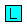\$B\$O(B each Line \$B\$N(B Leaders \$B\$r<(\$7(B,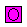\$B\$OB>\$N%@%s%5!<\$r<(\$7\$^\$9!%(B\$B\$G<(\$5\$l\$??M\$O(B, 1 x 4 \$B\$G(B\$B\$N?M\$HF1\$88~\$-\$G\$J\$1\$l\$P\$I\$3\$r8~\$\$\$F\$\$\$F\$b\$+\$^\$\$\$^\$;\$s!%(B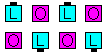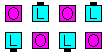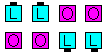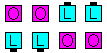Leader \$B\$,(B\$B1&\$N(B Wave \$B\$N(B setup... \$B:8\$N(B Wave \$B\$N(B setup... \$B1&\$N(B 2FL setup... \$B:8\$N(B 2FL setup.

Checker Board anything:
Each Line \$B\$N(B Leader (\$B\$N?M(B) \$B\$,\$*8_\$\$\$K(B Trade \$B\$r\$9\$k4V\$K(B, \$BB>\$N?M\$O(B anything call \$B\$r(B distorted 1 x 4 \$B\$G9T\$\$\$^\$9!%(B 2 x 4 \$B\$G=*\$o\$j\$^\$9!%(B

Checker Box anything:
Each Line \$B\$N(B Leader (\$B\$N?M(B) \$B\$,\$*8_\$\$\$K(B Trade \$B\$r\$9\$k4V\$K(B, \$BB>\$N?M\$O(B anything call \$B\$r(B distorted 2 x 2 \$B\$G9T\$\$\$^\$9!%(B 2 x 4 \$B\$G=*\$o\$j\$^\$9!%(B

\$BCm\$N?M(B (\$B>e\$N?^\$G(B\$B\$N?M(B) \$B\$O\$I\$3\$r8~\$\$\$F\$\$\$F\$b\$+\$^\$\$\$^\$;\$s!%(B \$B\$b\$7(B, Line \$B\$K(B Leader \$B\$,(B 3 \$B?M0J>e\$\$\$k\$J\$i(B, \$B%3!<%i!<\$O(B 'Prefer the anyone to Trade, all Checker Board | Box anything' \$B\$N\$h\$&\$K8@\$C\$F(B, Trade \$B\$9\$k?M\$r;X<(\$7\$J\$1\$l\$P\$J\$j\$^\$;\$s!%(B

Checker Board Recycle \$B\$H(B Checker Box Recycle \$B\$NBPHf!%(B

Checker Board Recycle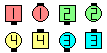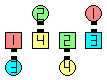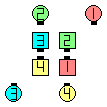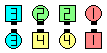Checker Board Recycle\$B\$NA0(B Leaders Tradeas Trailers make a 1 x 4\$B\$N8e(B Recycle\$B\$N8e(B \$B0LCV\$N:F9=@.(B\$B\$N8e(B \$B\$N8e(B

Checker Box Recycle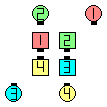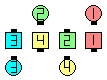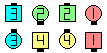Checker Box Recycle\$B\$NA0(B Leaders Tradeas Trailers make a 2 x 2\$B\$N8e(B Recycle\$B\$N8e(B \$B0LCV\$N:F9=@.(B\$B\$N8e(B \$B\$N8e(B

Note: Checker Board | Box is often taught as: Leaders Trade as the Others move into the Center and make the given formation (a 1 x 4 if Checker Board, or a 2 x 2 if Checker Box), do the anything call and then get back to the vacated spots. As dancers, we prefer to do the anything call working in a Distorted Column | Line | Wave | Box. That is, try to stay on the original spots and only move off them if necessary. For example, from Parallel L-H Waves, if the call is Checker Box Cross & Turn, the Trailing End (Belle) would simply U-Turn Back instead of move into the Center, make a Box, U-Turn Back, and Veer out to become an End.

Checker Board Dixie Style To A Wave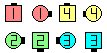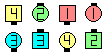Checker BoardDixie Style To A Wave\$B\$NA0(B Leaders in Lines Tradeas Others Distorted ColumnDixie Style To A Wave\$B\$N8e(B (\$B=*\$o\$j(B)

Checker formation anything [C4] : Leaders \$B\$O(B (Line \$B\$K\$\$\$k\$3\$H(B) \$B\$*8_\$\$\$K(B Trade \$B\$r\$7(B, \$BB>\$N?M\$O(B anything call \$B\$r(B distorted formation \$B\$G9T\$\$\$^\$9!%(B \$B;X<(\$5\$l\$k(B formation \$B\$O(B Diamond, Single 1/4 Tag, "Z" \$B\$N\$h\$&\$J(B 4 \$B?M\$N(B formation \$B\$G\$9!%(B \$B\$7\$?\$,\$C\$F(B, Checker Board \$B\$O(B Checker Column, Checker Line, Checker Wave \$B\$H\$b%3!<%k\$5\$l\$^\$9!%(B

Checker Diamond Cut The Diamond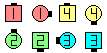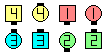Checker DiamondCut The Diamond\$B\$NA0(B Leaders in Lines Tradeas Others Distorted DiamondCut The Diamond\$B\$N8e(B (\$B=*\$o\$j(B)